# Overall Mass Transfer Coefficients Notes | Study Mass Transfer - Chemical Engineering

## Chemical Engineering: Overall Mass Transfer Coefficients Notes | Study Mass Transfer - Chemical Engineering

The document Overall Mass Transfer Coefficients Notes | Study Mass Transfer - Chemical Engineering is a part of the Chemical Engineering Course Mass Transfer.
All you need of Chemical Engineering at this link: Chemical Engineering

3.6.2 Overall mass transfer coefficients
Experimentally the mass transfer film coefficients ky and kx are difficult to measure except for cases where the concentration difference across one phase is small and can be neglected. Under these circumstances, the overall mass transfer coefficients Ky and Kx are measured on the basis of the gas phase or the liquid phase. The entire two-phase mass transfer effect can then be measured in terms of gas phase molar fraction driving force as: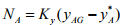(3.76)
where, Ky is based on the overall driving force for the gas phase, in mole/m2.s and y*A  is the value of concentration in the gas phase that would be in the equilibrium with xAL. Similarly, the entire two-phase mass transfer effect can then be measured in terms of liquid phase molar fraction driving force as: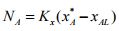(3.77)
where Kx is based on the overall driving force for the liquid phase, in mole/m2.s and x*A  is the value of concentration in the liquid phase that would be in the equilibrium with yAG. A relation between the overall coefficients and the individual mass transfer film coefficients can be obtained when the equilibrium relation is linear as yAi = mxAi . The linear equilibrium condition can be obtained at the low concentrations, where Henry’s law is applicable. Here the proportionality constant m is defined as m= H/P. Utilizing the relationship, yAi =mxAi   , gas and liquid phase concentrations can be related by
y*A = mxAL                                                          (3.78)
and
yAG = mx*A                                                  (3.79)

Rearranging Equation (3.76), one can get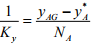(3.80)
From geometry, yAG - y*A  can be written as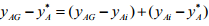(3.81)

Substituting Equation (3.81) in Equation (3.80)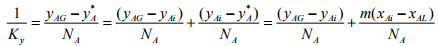(3.82)
The substitution of Equation (3.76) into the Equation (3.82) relates overall gas phase mass transfer coefficient (Ky) to the individual film coefficients by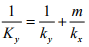(3.83)
Similarly the relation of overall liquid phase mass transfer coefficient (Kx) to the individual film coefficients can be derived as follows: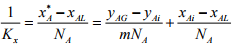(3.84)

Or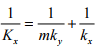(3.85)
The following relationships between the mass transfer resistances can be made from the Equations (3.83) and (3.85):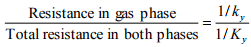(3.86)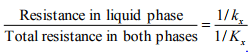(3.87)
If solute A is very soluble in the liquid, m is very small. Then the term m/kx in Equation (3.83) becomes minor and consequently the major resistance is represented by 1/ky. In this case, it is said that the rate of mass transfer is gas phase controlled. In the extreme it becomes: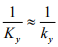(3.88)
The total resistance equals the gas film resistance. The absorption of a very soluble gas, such as ammonia in water is an example of this kind. Conversely when solute A is relatively insoluble in the liquid, m is very large. Consequently the first term of Equation (3.85) becomes minor and the major resistance to the mass transfer resides within the liquid. The system becomes liquid film controlling. Finally this becomes: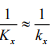(3.89)
The total resistance equals the liquid film resistance. The absorption of a gas of low solubility, such as carbon dioxide or oxygen in water is of this type of system.

Example problem 3.3:
In an experimental study of the absorption of ammonia by water in a wetted-wall column, the value of overall mass transfer coefficient, KG was found to be 2.75 x 10-6 kmol/m-s-kPa. At one point in the column, the composition of the gas and liquid phases were 8.0 and 0.115 mole% NH3, respectively. The temperature was 300K and the total pressure was 1 atm. Eighty five % of the total resistance to mass transfer was found to be in the gas phase. At 300 K, Ammonia –water solutions follows Henry’s law upto 5 mole% ammonia in the liquid, with m = 1.64 when the total pressure is 1 atm. Calculate the individual film coefficients and the interfacial concentrations. Interfacial concentrations lie on the equilibrium line.

Solution 3.3:
The first step in the solution is to convert the given overall coefficient from KG to Ky.
Ky = KG P = 2.75 x 10-6 x 101.3 = 2.786 x 10-4 kmol/m2-s

For a gas-phase resistance that accounts for 85% of the total resistance,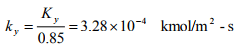From Equation,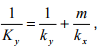by substituting the values of Ky , ky and m
kx = 3.05 x 10-3 kmol/m2-s

To estimate the ammonia flux and the interfacial concentrations at this particular point in the column use the equation, y*A = mxA,L to calculate
y*A = mxA,L = 1.64 x 1.15 x 10-3 = 1.886 x 10-3
The flux is from equation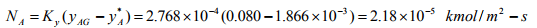Calculate the gas-phase interfacial concentration from equation,
Na = ky( yAG - yA,i) as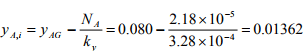Since the interfacial concentrations lie on the equilibrium line,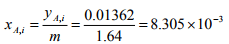Nomenclature

a  Cross-sectional area [m2 ]
s  Fraction of surface renewed/unit time [-]
C  Molar concentration [mol/m3 ]
Sav Average cross-sectional area for diffusion [m2 ]
d  Diameter [m]
T  Temperature [K]
dp  Diameter of a particle [m]
t  Time [s]
DAB    Diffusivity of A in B [m2/s]
u  Velocity [m/s]
DE  Eddy diffusivity [m2/s]
U  average velocity [m/s]
DK  Knudsen diffusion coefficient [m2/s]
Ua  Free stream velocity [m/s]
DS  Surface diffusion coefficient [m2/s]
V   Volume [m3 ]
ED  Activation energy [J/mol]
w  Mass fraction [-]
Gm  Molar mass velocity [mol/m2.s]
W  Mass transfer rate [mol/s]
Gy  Mass velocity of gas [kg/m2.s]
x  Mole fraction for liquid [-]
ΔHVA  Latent heat of vaporization of component A [J/mol]
y  Mole fraction for gas [-]
J  Flux based on arbitrary
X,Y, Z  Coordinates
K  Proportionality constant defined in Equation (1.79) [-]
x*,y* Equilibrium mole fraction of solute in liquid and gas phase,respectively [-]
K  Overall mass transfer coefficient [m/s]
φ  Association factor [-]
k/,k Individual mass transfer coefficient [m/s]
ε     Porosity [-] l  Length [m]
v  Molar volume [mol/m3 ]
m  Mass [kg]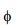Packing fraction [-]
M  Molecular weight
σAB   Characteristic length parameter of binary mixture of A and B [m]
N  Flux [mol/m2.s]
τ  Tortuosity [-]
p   Partial pressure [N/m2 ]
Ω  collision integral [-]
P  Total pressure [N/m2 ]
ρ  Density [kg/m]
PVVapor pressure of A [N/m2 ]
δ  Film thickness [m]
μ Viscosity [kg/m.s]
R   Universal gas constant [J/mol.K]

The document Overall Mass Transfer Coefficients Notes | Study Mass Transfer - Chemical Engineering is a part of the Chemical Engineering Course Mass Transfer.
All you need of Chemical Engineering at this link: Chemical EngineeringUse Code STAYHOME200 and get INR 200 additional OFF

## Mass Transfer

29 videos|45 docs|44 tests

Track your progress, build streaks, highlight & save important lessons and more!

,

,

,

,

,

,

,

,

,

,

,

,

,

,

,

,

,

,

,

,

,

;